﻿ Oblique shock - XSim

# Oblique shock

Update: December 17, 2018
OpenFOAM 4.x

## Case directory

\$FOAM_TUTORIALS/compressible/rhoCentralFoam/obliqueShock

## Summary

A virtual non-viscous gas flow, "normalized" to have a sound velocity of 1 m/s at a temperature of 1 K, flowing in from two directions and colliding each other, is calculated for 10 seconds.

The gas flows in from the region "inlet" at 2.9 m/s (Mach number 2.9) and the region "top" at (2.61933, -0.50632) m/s (Mach number about 2.66), and after colliding, flows out from the region "outlet". The region "bottom" is set to a symmetric boundary.Model geometry

The turbulence model is not used, and the analysis is performed in 2-dimensions.

The physical properties of the gas are specified in the file constant/thermophysicalProperties as follows.

```mixture
{
specie
{
nMoles          1;
molWeight       11640.3;
}
thermodynamics
{
Cp              2.5;
Hf              0;
}
transport
{
mu              0;
Pr              1;
}
}
```

The meshes are as follows, and the number of mesh is 1800.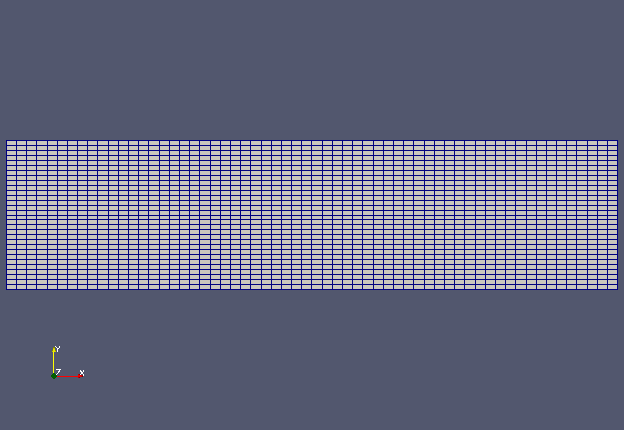Meshes

The calculation result is as follows.

The flow velocity is as follows.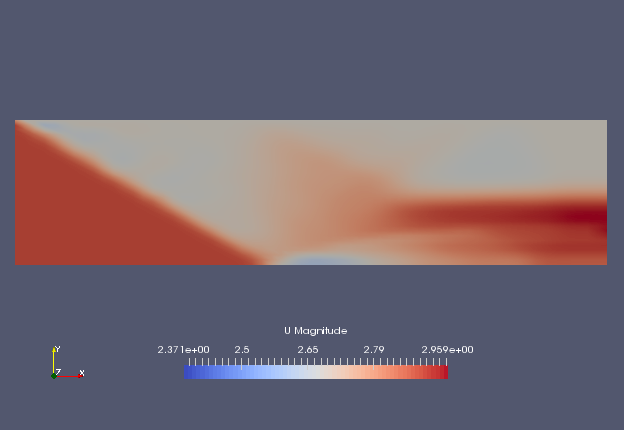Flow velocity at 1 sec (U)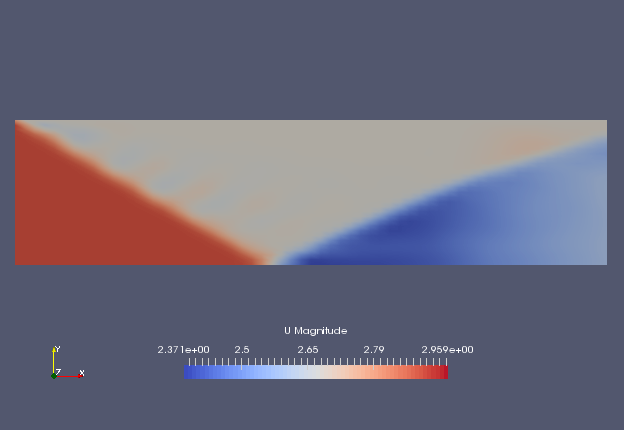Flow velocity at 2 sec (U)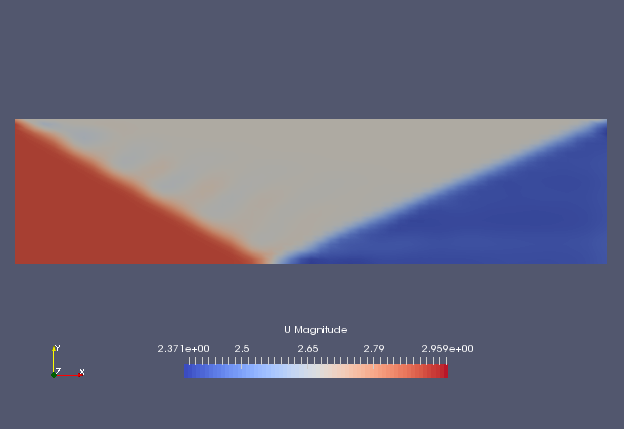Flow velocity at 10 sec (U)

And the pressure is as follows.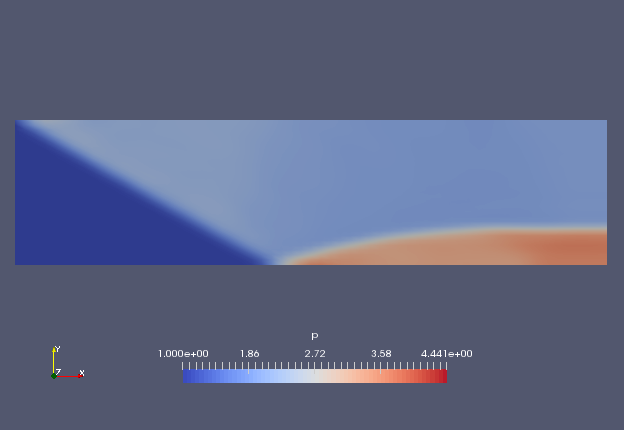Pressure at 1 sec (p)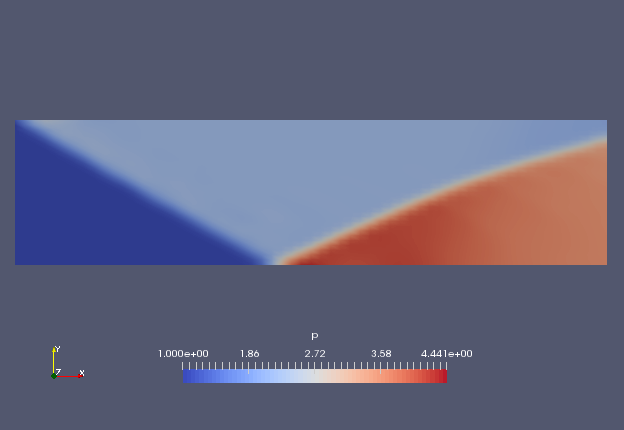Pressure at 2 sec (p)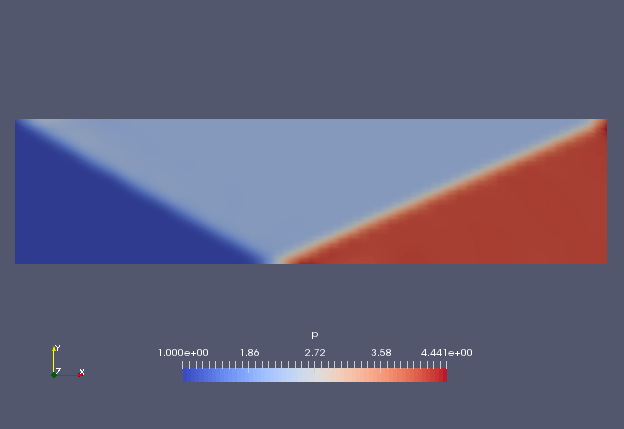Pressure at 10 sec (p)

## Commands

cp -r \$FOAM_TUTORIALS/compressible/rhoCentralFoam/obliqueShock obliqueShock
cd obliqueShock

blockMesh
rhoCentralFoam

paraFoam

## Calculation time

7.12 seconds *Single, Inter(R) Core(TM) i7-8700 CPU @ 3.20GHz 3.19GHz